# 福布斯系列(5)-数据清洗(a)

projects-forbes Pandas Numpy

## 1 前言

• windows7
• jupyter notebook

## 2 数据清洗的目的

1. 替换NaN值
2. 将字符串型数字转换为数字类型
3. 将数字后面多余的字母等文字剔除
4. 将公司和国家进行拆分
5. 将国家名称进行统一
6. 按列名将DataFrame重新排序

年份                    int64

dtype: object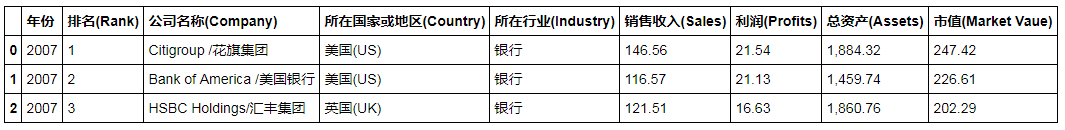Year               int64
Rank               int64
Company_cn_en     object
Company_en        object
Company_cn        object
Country_cn_en     object
Country_cn        object
Country_en        object
Industry_cn       object
Industry_en       object
Sales            float64
Profits          float64
Assets           float64
Market_value     float64
dtype: object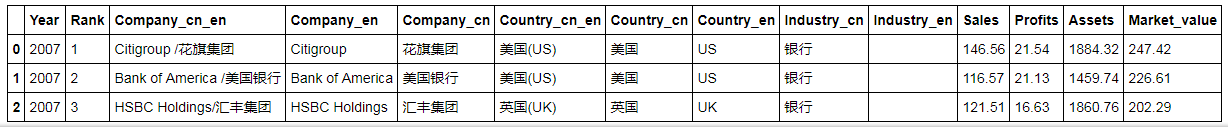## 3 数据清洗的详细过程

import pandas as pd
import numpy as np



2007年的数据，原始数据的单位为十亿美元。

### 3.1 先查看数据的类型（dtype）和结构

df_2007 = pd.read_csv('./data/data_forbes_2007.csv', encoding='gbk', thousands=',')
print('the shape of DataFrame: ', df_2007.shape)
print(df_2007.dtypes)

out:
the shape of DataFrame:  (2000, 9)

dtype: objectcolumn_update = ['Year', 'Rank', 'Company_cn_en', 'Country_cn_en',
'Industry_cn', 'Sales', 'Profits', 'Assets', 'Market_value']
df_2007.columns = column_update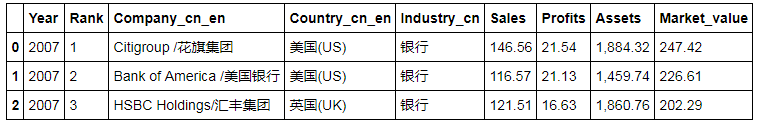### 3.2 将字符串转换为数字类型

df_2007[df_2007['Sales'].str.contains('.*[A-Za-z]', regex=True)]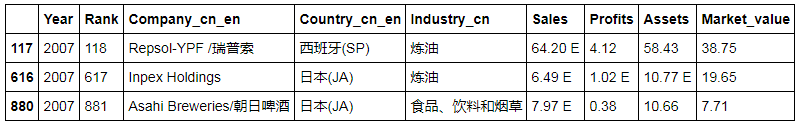df_2007['Sales'] = df_2007['Sales'].replace('([A-Za-z])', '', regex=True)


df_2007.loc[[117,616,880], :]df_2007[df_2007['Assets'].str.contains('.*[A-Za-z]', regex=True)]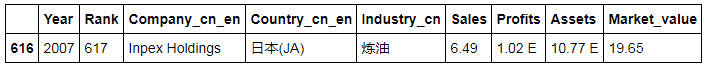# 将数字后面的字母进行替换
df_2007['Assets'] = df_2007['Assets'].replace('([A-Za-z])', '', regex=True)

# 千分位数字的逗号被识别为string了，需要替换
df_2007['Assets'] = df_2007['Assets'].replace(',', '', regex=True)
df_2007.loc[616, :]

out:
Year                       2007
Rank                        617
Company_cn_en    Inpex Holdings
Country_cn_en            日本(JA)
Industry_cn                  炼油
Sales                     6.49
Profits                  1.02 E
Assets                   10.77
Market_value              19.65
Name: 616, dtype: object


df_2007[pd.isnull(df_2007['Profits'])]

# 将NaN值替换为0
df_2007['Profits'].fillna(0, inplace=True)
df_2007.loc[[958,1440,1544,1912], :]df_2007['Profits'] = df_2007['Profits'].replace('([A-Za-z])', '', regex=True)
df_2007.loc[[117,616,880], :]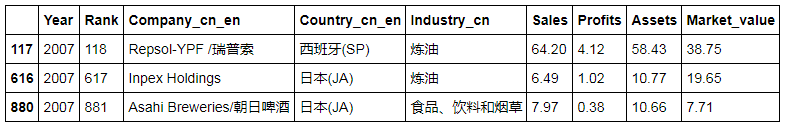df_2007['Sales'] = pd.to_numeric(df_2007['Sales'])
df_2007['Profits'] = pd.to_numeric(df_2007['Profits'])
df_2007['Assets'] = pd.to_numeric(df_2007['Assets'])
df_2007.dtypes

out:
Year               int64
Rank               int64
Company_cn_en     object
Country_cn_en     object
Industry_cn       object
Sales            float64
Profits          float64
Assets           float64
Market_value     float64
dtype: object


### 3.3 拆分”Company_cn_en”列

df_2007['Company_en'],df_2007['Company_cn'] = df_2007['Company_cn_en'].str.split('/', 1).str
print(df_2007['Company_en'][:5])
print(df_2007['Company_cn'] [-5:])
df_2007.tail(3)

out:
0           Citigroup
1     Bank of America
2        HSBC Holdings
3    General Electric
4      JPMorgan Chase
Name: Company_en, dtype: object
1995    NaN
1996    NaN
1997    NaN
1998    NaN
1999    NaN
Name: Company_cn, dtype: object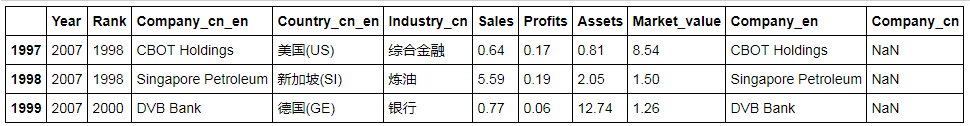### 3.4 拆分”Country_cn_en”列

df_2007['Country_cn'],df_2007['Country_en'] = df_2007['Country_cn_en'].str.split('(', 1).str
print(df_2007['Country_cn'][:5])
print(df_2007['Country_en'][-5:])

out:
0    美国
1    美国
2    英国
3    美国
4    美国
Name: Country_cn, dtype: object
1995    US)
1996    US)
1997    US)
1998    SI)
1999    GE)
Name: Country_en, dtype: object


df_2007['Country_en'] = df_2007['Country_en'].str.slice(0,-1)


• 中国大陆：CN；
• 中国香港：CN-HK；
• 中国台湾：CN-TA。
# 查找含“中国”的数据
df_2007[df_2007['Country_cn'].str.contains('中国',regex=True)]

# 替换并查看结果
df_2007['Country_en'] = df_2007['Country_en'].replace(['HK.*','TA'],['CN-HK', 'CN-TA'],regex=True)
df_2007[df_2007['Country_en'].str.contains('CN',regex=True)]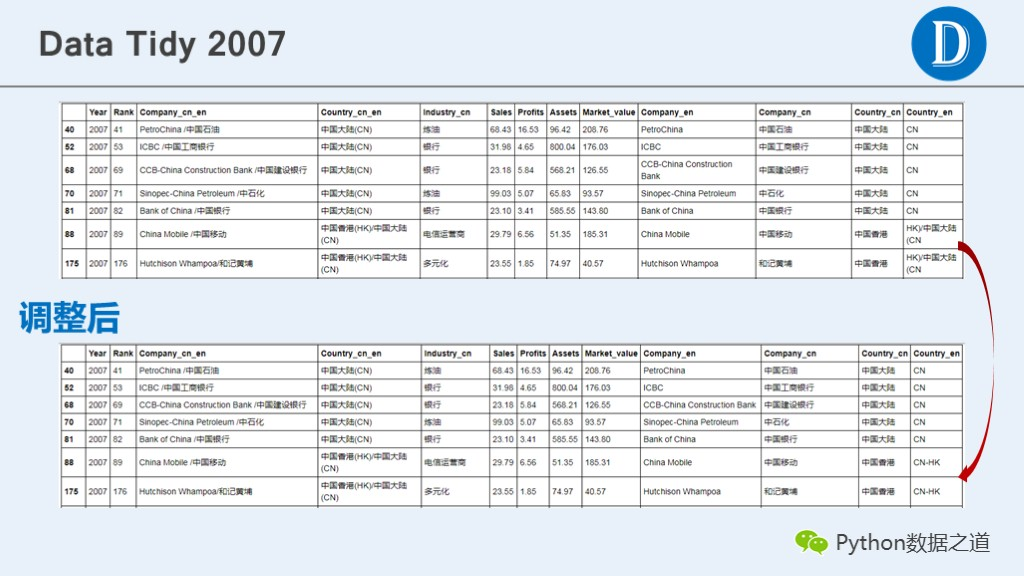df_2007['Industry_en'] = ''
df_2007.tail(3)


### 3.5 将列名进行重新排序

columns_sort = ['Year', 'Rank', 'Company_cn_en','Company_en',
'Company_cn', 'Country_cn_en', 'Country_cn',
'Country_en', 'Industry_cn', 'Industry_en',
'Sales', 'Profits', 'Assets', 'Market_value']

# 按指定list重新将columns进行排序
df_2007 = df_2007.reindex(columns=columns_sort)
print(df_2007.shape)
print(df_2007.dtypes)

out:
(2000, 14)
Year               int64
Rank               int64
Company_cn_en     object
Company_en        object
Company_cn        object
Country_cn_en     object
Country_cn        object
Country_en        object
Industry_cn       object
Industry_en       object
Sales            float64
Profits          float64
Assets           float64
Market_value     float64
dtype: object## 4 总结